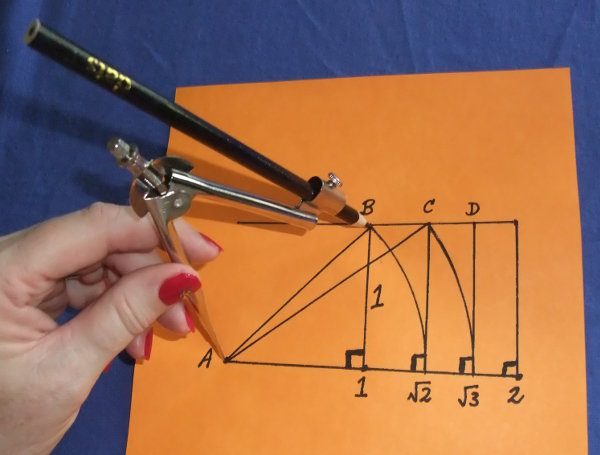# Surds

What is a surd?

When the square root of a number is a decimal number (and not a whole number), it is called a surd.
Examples of surds are:

• 2 = 1.4
• 3 = 1.7
• 5 = 2.2
• 6 = 2.4
• 7 = 2.6

What is NOT a surd?

When a square root of a number is a whole number, it is not a surd.
Examples that are not surds are:

• 1 = 1
• 4 = 2
• 9 = 3

## Maths Fun - Compass Activity• Draw a right-angled triangle. Make each of the sides that form the right angle 1 unit long.
• Using Pythagoras's Rule, the hypotenuse is 2.
• Extend a compass to the length of 2 and draw the next right-angled triangle with a side of 1 and the second side 2.
• Using Pythagoras's Rule, the hypotenuse of the next triangle is 3.
• Continue this to find the square roots of whole numbers.
• Consider that the ancient Greeks probably used this method to work square roots of surds and non-surds. Clever, isn't it?

## Questions - Which are Surds?

Find the square roots of these numbers - 1, 2, 3, 4, 5, 25, 50, 100.
Which are surds?

Surds are the square roots of 2, 3, 5, 50.

## Example One - Simplify Surds

Simplify:
(a) 8
(b) 27
(c) 48
(d) 50

Firstly, find the factors of the number where one of the factors is a square number such as 4, 9, 16, 25 and so on. Then simplify.

(a) 8 = 4 × 2 = 22
(b) 27 = 9 × 3 = 33
(c) 48 = 16 × 3 = 43
(d) 50 = 25 × 2 = 52

(a) 12
(b) 45
(c) 500
(d) 75

(a) 23
(b) 35
(c) 105
(d) 53

## Example Two - Entire Surds

Make these entire surds:
(a) 32
(b) 25
(c) 35
(d) 57

This is the reverse of Example One above.

(a) 32 = 9 × 2 = 18
(b) 25 = 4 × 5 = 20
(c) 35 = 9 × 5 = 45
(d) 57 = 25 × 7 = 175

(a) 53
(b) 210
(c) 37
(d) 73

(a) 75
(b) 40
(c) 63
(d) 147

## Example Three - Simplify these Multiplied Surds

Simplify these surds:
(a) 23 × 53
(b) 25 × 52

(a) 23 × 53
= 4 × 3 × 25 × 3
= 900
= 30

(b) 25 × 52
= 4 × 5 × 25 × 2
= 1000
= 100 × 10
= 1010

## Example Four - Simplify these Divided Surds

Simplify these surds:

 (a) 85 25
 (b) 67 × 53 23

 (a) 85 = 8 = 4 25 2
 (b) 67 × 53 = 67 × 5 = 37 × 5 = 157 23 2

## Questions - Simplify these Surds

(a) 23 × 53

 (b) 43 26

(a) 30
(b) 2

## Example Five - Simplifying Surds on the Bottom of a Fraction

Simplify these surds:

 (a) 3 2
 (b) 5 3

If there is a surd on the bottom of a fraction, multiply both the top and the bottom of the fraction by that surd. Then simplify.

 (a) 3 = 32 = 32 2 2 × 2 2
 (b) 5 = 53 = 53 3 3 × 3 3

## Questions - Simplify these Surds

 (a) 7 2
 (b) 8 2